Question

# 10. A multiple-choice test has 30 questions and each one has five possible answers, of which one...

10. A multiple-choice test has 30 questions and each one has five possible answers, of which one is correct. If all answers were guesses, find the probability ofgetting exactly four correct answers. (Points : 3)
.0604
.1325
.2552
.8000

n=30

We are having 5 choices. This means that p=1/5.

Using Binomial Distribution

P[X=4]=30C4 *(0.2)^4*(1-0.2)^(30-4)=0.132522

#### Earn Coins

Coins can be redeemed for fabulous gifts.

Similar Homework Help Questions
• ### statistics

A multiple-choice test has 30 questions and each one has five possible answers, of which one is correct. If all answers were guesses, find the probability of gettingexactly four correct answers. (Points : 3).0604.1325.2552.8000

• ### Probability

A multiple-choice test has 30 questions and each one has five possible answers, of which one is correct. If all answers were guesses, find the probability of gettingexactly four correct answers. (Points : 3).0604.1325.2552.8000

• ### A multiple choice test has 10 questions each of which has 4 possible answers, only one...

A multiple choice test has 10 questions each of which has 4 possible answers, only one of which is correct. If Judy, who forgot to study for the test, guesses on all questions, what is the probability that she will answer exactly 3 questions correctly?

• ### A multiple choice test has 30 questions. Each questions five possible answers, of which one is...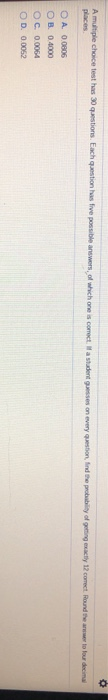A multiple choice test has 30 questions. Each questions five possible answers, of which one is correct a studentesses on every question in the probability of ging eactly 12 corect Round them to four decimal O A 0.0806 OB 0.4000 OC. 0.0064 OD. 0.0052

• ### A multiple choice test consists of 60 questions. each question has 4 possible answers of which...

A multiple choice test consists of 60 questions. each question has 4 possible answers of which one is correct. If all answers are random guesses, estimate the probability that of 140 randomly selected seeds, exactly 100 will germinate

• ### Find the indicated probability, A multiple choice test has 8 questions each of which has 4...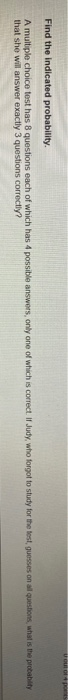Find the indicated probability, A multiple choice test has 8 questions each of which has 4 possible answers, only one of which is correct. Il Judy, who forgot to study for the test guesses on all questions, what is the probability that she will answer exactly 3 questions correctly?

• ### Selve the problem. (Spts.) 9) Multiple-choice questions on a test each have 6 possible answers, one...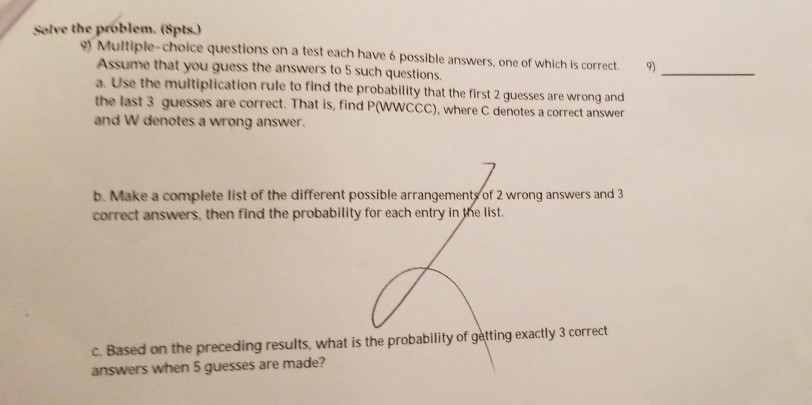Selve the problem. (Spts.) 9) Multiple-choice questions on a test each have 6 possible answers, one of which is correct. Assume that you guess the answers to 5 such questions. a. Use the multiplication rule to find the probability that the first 2 guesses are wrong and the last 3 guesses are correct and W denotes a wrong answer 9) . That is, find P(WWCCC), where C denotes a correct answer b. Make a complete list of the different possible...

• ### A multiple choice test has 25 questions, each with 5 possible choices, exactly one of which...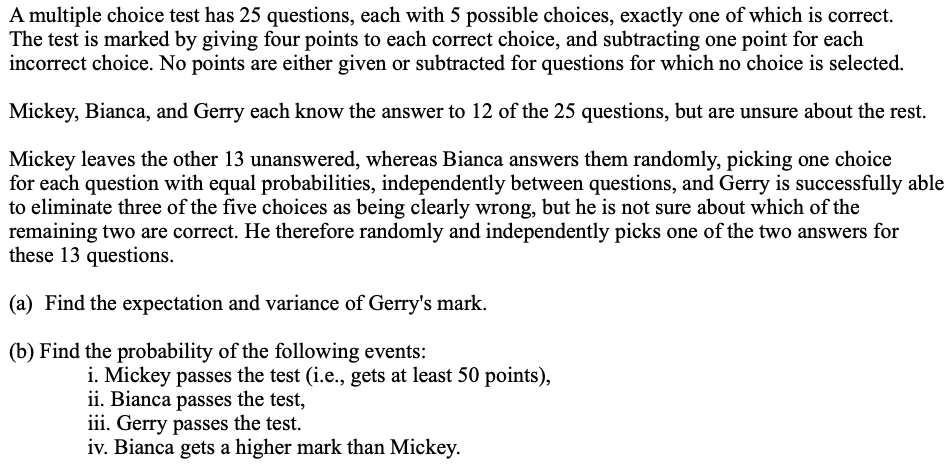A multiple choice test has 25 questions, each with 5 possible choices, exactly one of which is correct. The test is marked by giving four points to each correct choice, and subtracting one point for each incorrect choice. No points are either given or subtracted for questions for which no choice is selected. Mickey, Bianca, and Gerry each know the answer to 12 of the 25 questions, but are unsure about the rest. Mickey leaves the other 13 unanswered, whereas...

• ### Multiple-choice questions each have five possible answers (a, b, c, d, e), one of which is...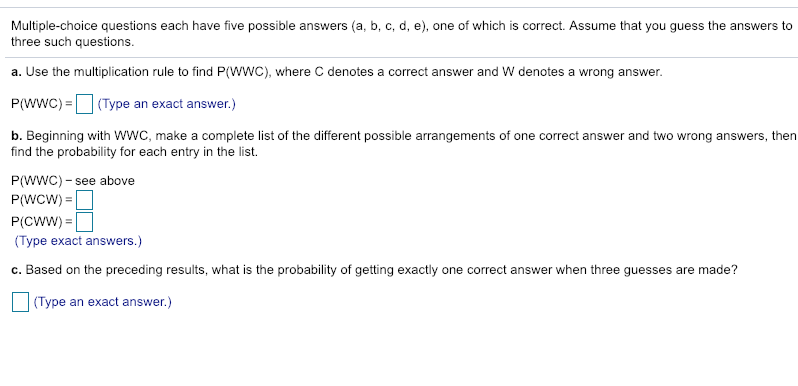Multiple-choice questions each have five possible answers (a, b, c, d, e), one of which is correct. Assume that you guess the answers to three such questions a. Use the multiplication rule to find P(WWC), where C denotes a correct answer and W denotes a wrong answer. P(WWC) (Type an exact answer.) b. Beginning with WWC, make a complete list of the different possible arrangements of one correct answer and two wrong answers, then find the probability for each entry in the...

• ### 5) A student takes a multiple choice test with 12 questions, Each question has 4 possible...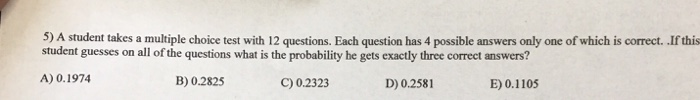5) A student takes a multiple choice test with 12 questions, Each question has 4 possible answers only one of which is correct. .If this student guesses on all of the questions what is the probability he gets exactly three correct answers? A) 0.1974 C) 0.2323 B) 0.2825 D) 0.2581 E) 0.1105